Anesthesia Pharmacology: Partial Pressures

• As noted earlier, the pressure in a tank of gas will be dependent on  the temperature (molecular velocities) and mass.

• If we have a gas mixture the question is what is the nature of the contribution of each different molecule type to the pressure exerted on the wall of the tank.

• It turns out to the pressure which might be attributed to one particular type of molecule is not dependent on whether or the other molecule type is present or not.

• 1This observation is  called Dalton's Law of partial pressures which "states that in a mixture of gases the pressure exerted by each gas is the same as that which it would exert if it alone occupied the container."

• Using Dalton's law in combination with Boyle's Law, the partial pressure of the gas  in a mixture can be determined by taking the total pressure and multiplying it by the fractional concentration of the particular gas.

• 1An example would be the case in which a cylinder exhibits in ambient pressure of 100 kPa which which would reflect a case in which 50% of the mixture is nitrous oxide and the other 50% is oxygen.

• So, according to Dalton's law the pressure that would be exerted by the nitrous oxide would-be independent of the presence of oxygen, in other words the same as if it were alone in the cylinder.

• If, however it were alone in the cylinder it would be occupying all the volume, not just one-half of the cylinder.  Boyle's Law would indicate that in order for V2  = 2 x V1 , there would have to be a corresponding decrease in cylinder pressure from 100 kPa to 50 kPa. P1 x V1 = P2 x V2 or P2 =  (P1 x V1 ) / V2 , since V1 /V2 = 1/2 then P2 = 1/2 P1 or P2 = 50 kPA

• 1Again let's consider a cylinder, in this case containing air at a pressure of 100 kPa.

• Furthermore, the percentage of oxygen is about 21% and the percentage of nitrogen is about 79%.  Given that we start with 21% oxygen than oxygen's contribution to the pressure would be 21% x 100 kPa  or 21 kPa.

• Similarly, the nitrogen pressure would be about 79 kPa.

• We can readily convert from kPa units to mm Hg by recognizing that 100 kPa is about 750 mm Hg.  Therefore, oxygen contribution to the total pressure of 21 kPa could be expressed as about 157 mm Hg, whereas nitrogen's contribution would be about 593 mm Hg.

• We often use the expression "a gas's partial pressure" This designation refers to the pressure that each gas in a mixture will exert, which is its partial pressure.  This consideration is independent of what gases might be involved.  For example, if we started out with a certain number of moles of helium gas and then doubled it, we would know from the PV = nRT relationship that the pressure would double.  However, suppose it that instead of doubling the number of helium gas moles we add another gas instead, such as argon. we would note that the pressure would still double, because each gas contributes 50% such that the total pressure equals the partial pressure provided by helium + the partial pressure provided by argon. So this conclusion doesn't depend on what gas is actually used provided that the gases do not chemically interact.

• In the clinical setting, that is, with respect to the delivery of anesthetic gases, is important in considering the water vapor in the partial pressure calculation.

• The particular example would be for the case in which the end-tidal CO2 concentration as a dry gas is measured as 5.6%.  If the ambient pressure were 101.3 kPa an error would be introduced in finding the alveolar CO2  (PACO2) if we only multiplied 5.6% x 101.3 kPa and did not take into account that the ambient pressure includes a water vapor pressure of about 6.3 kPa.

• The water vapor concentration must first be subtracted from the ambient pressure (101.3 kPa - 6.3 kPa) prior to multiplying by 5.6%.  [5.6% x 95 kPa = 5.3 kPa which would correspond to about 40 mm Hg.]

• In the relationship, PV = nRT, n is referred to as the number of moles.  In chemistry, and even more general than that, a large number of molecules is referred to in terms of a standardized number which is 6.02 x 1023 molecules or 1 mole. Therefore, the number of molecules are represented in terms of number of moles.

• The mole is defined so that the mass of a mole of carbon atoms -the carbon 12 isotope is exactly 12 grams.  The carbon 12 isotope consists of six protons and six neutrons.

• 6.02 x 1023 molecules is also known as Avagadro's number.

• In addition, one mole of any gas at standard temperature and pressure occupies 22.4 l. Recall from the gas calculator (first page in the physics section) that a pressure of 1 atmosphere and a temperature of 273oK, one mole will correspond to 22.4 l.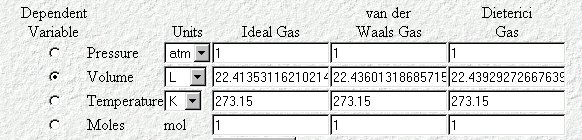• Therefore, 32 grams of oxygen or 44 grams of carbon dioxide or two grams of hydrogen (a mole's worth in each case) would correspond to 22.4 L

Periodic Table of the Elements (click)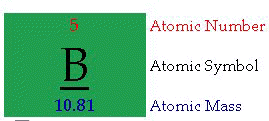• Atomic Number:  "The number of protons in an atom defines what element it is. For example carbon atoms have six protons, hydrogen atoms have one, and oxygen atoms have eight. The number of protons in an atom is referred to as the atomic number of that element. The number of
protons in an atom also determines the chemical behavior of the element."

• Atomic Symbol: "The atomic symbol is one or two letters chosen to represent an element ("H" for "hydrogen," etc.). These symbols are used
internationally. Typically, a symbol is the truncated name of the element or the truncated Latin name of the element. Click here for a
list of the elements and their symbols."

• Atomic Mass:  "The atomic mass is the average mass of an element in atomic mass units ("amu"). Though individual atoms always have an integer number of amus, the atomic mass on the periodic table is stated as a decimal number because it is an average of the various isotopes
of an element. Isotopes can have a weight either more or less than the average. The average number of neutrons for an element can
be found by subtracting the number of protons (atomic number) from the atomic mass."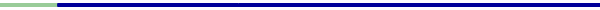: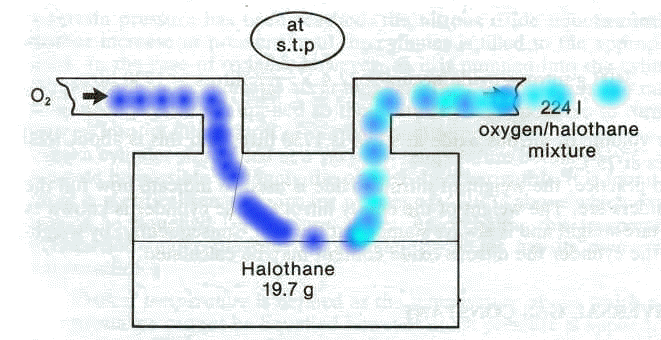• Pictured above is a representation of the vaporizer.

• Most anesthetic "gases" are in fact volatile liquids and therefore must be vaporized in order to be delivered in a gas form to the lungs.  (Nitrous oxide is an exception since it is a gas at room temperature).

• Given that halothane (Fluothane) would normally be a liquid, the addition of heat which would increase the kinetic energy of the halothane (Fluothane) molecules would be required in order to promote vaporization.

• If the temperature is held constant, then a steady state or equilibrium condition will be reached in which the concentration of the gas molecules remain constant on average.  [This condition really reflects the approximate equivalence of molecules moving from the liquid phase into the gas phase and from the gas phase back into the liquid phase] The pressure exerted by the vaporized molecules on the walls of the container would be referred to as the vapor pressure.

• 2So, the particular problem is to determine the halothane (Fluothane) percentage concentration in oxygen shown flowing into the vaporizer completely vaporizes the 19.7 g of halothane (Fluothane) present in the vaporizer.

• Conveniently, the molecular weight of halothane (Fluothane) is 197; therefore, by definition 197 grams of halothane (Fluothane) = 1 mole.

• We also know that one mole will occupy 22.4 l (at stp).

• We're going to vaporize this 19.7 g of halothane (Fluothane) into a total volume of 224 liters (what a coincidence).  Now 19.7 g of halothane (Fluothane) is equal to 0.1 moles.

• Since one mole will occupy 22.4 l, 0.1 moles will occupy 2.24 l. If the total volume had been 2.24 l, than the halothane (Fluothane) concentration would be 100%.

• However, as noted earlier the total volume is 224 liters and therefore the halothane (Fluothane) concentration is obtained by dividing 2.24 liters by 224 liters, which is equal to 1%

• 2Another example has to do with a nitrous oxide cylinder, which is known to contain 3.4 kg of nitrous oxide (N2O), which has a molecular weight of 44; therefore, 44 grams of nitrous oxide = 1 mole [From the periodic chart, we get the molecular weight using the formula  (N2O), by adding 14 for nitrogen + 16 for oxygen (twice)].

• The question is, assuming standard temperature and pressure, what would be the volume of nitrous oxide that might be obtained from the cylinder.

• Now we know that 44 g nitrous oxide (one  mole) will occupy 22.4 l, but we have not 44 g but 3400 g nitrous oxide.  Therefore the answer would be given by multiplying 3400/44 x 22.4 liters = 1730 l.

• To find out the volume at a different temperature we would simply use Boyle's Law -- for example at 15oC, one would obtain about 1825 l.

• Before we continue and talk more about vaporizer action, let's consider why  in this particular example above the vaporizer is emptied of halothane (Fluothane).

• Now we may have added heat in order to facilitate vaporization.  For a moment must consider the case in which there is no oxygen flow through the vaporizer.

• The addition of some heat will result in an equilibrium in which on average a certain number of halothane (Fluothane) molecules will exist in the vapor phase and exert the vapor pressure.

• As noted earlier, the exact number of molecules vary somewhat and furthermore there is a flux of molecules (movement of molecules) from the liquid phase into the gas phase which is roughly balanced by another flux of molecules from the gas phase into the liquid phase.

•  In this condition, we have a relatively static condition and although the volume of liquid halothane (Fluothane) has been reduced, it has been reduced only slightly by the number of molecules in the gases.

• What the flow of oxygen does however is to prevent the attainment of such an equilibrium state because at any moment the concentration of halothane (Fluothane) molecules in the gas phase is reduced as they are carried out of the system by the oxygen flow.  The system cannot under the circumstances reach an equilibrium because we're constantly removing (reducing) halothane molecules from the gas phase.  Under the circumstances, there will be a significant net flux from the liquid phase to the gas phase which will ultimately deplete the halothane (Fluothane) reservoir. One last point is that in the usual mode of vaporizer action the volume out is larger than the volume in, since more molecules are added during the process, i.e. anesthetic molecules.

• 3The % concentration (F) of a gas flowing through vaporizer is given by:

• F = Anesthetic vapor pressure (mm Hg) / Ambient pressure (mm Hg); furthermore, the anesthetic vapor flow (cc/min) = (F/1-F) *fresh gas flow rate (cc/min.)

• For example, using isoflurane (Forane) as an example which has a vapor pressure at 20oC and 760 mm Hg of 239 mm Hg then the fraction (%) of isoflurane (Forane) output would be the ratio of the anesthetic vapor pressure (mm Hg) to the ambient pressure e.g. 760 mm Hg at sea level) or 239 mm Hg/760 mm Hg = 31%

• Applying this relationship: the anesthetic vapor flow (cc/min) = (F/1-F) *fresh gas flow rate (cc/min.) one obtains that the flow of isoflurane (Forane) vapor from the vaporizer is equal to 31%/69% X 5000 cc/minute where 5000 cc:/minute (5 liters/minute) is the input oxygen flow rate--the oxygen bubbling through the volatile agent..

• Solving the equation gives the results of 2250 cc/minute

• Since molecules of isoflurane (Forane) are added to the gases, to the process of vaporization, the total outflow gas from the vaporizer is the sum of the input volume and the volume contributed by isoflurane (Forane) vapor or  2250 cc/minute + 5000 cc/minute =7250 cc/minute.

• The relationship that describes the concentration of anesthetic output from the vaporizer and includes the diluent contribution would be as follows:

1. Concentration vapor = (Flowvaporizer x anesthetic vapor pressure)  / [atmospheric pressure x (Flowvaporizer + Flowdiluent) - (anesthetic vapor pressure x Flowdiluent)]

2. Using the above example for isoflurane (Forane) and setting the Flowdiluent= 70,000 cc:/minute we would obtain:

3. Concentration vapor = (7250 cc/min x 239 mmHg) / [760 mmHg x (7250 cc/min + 70000 cc/min)] - (239 mmHg x 70000 cc/min) which is about 4%.

4. Concentration vapor is also about equal to the vaporizer anesthetic output / total flow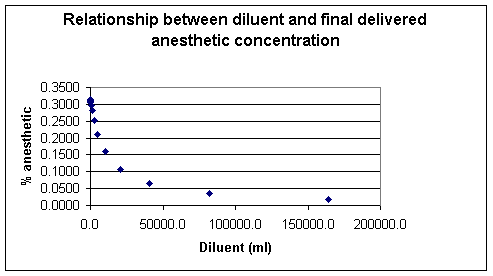• The vapor pressure is a characteristic of the particular molecules and will be dependent on the ambient temperature.

• Generally the vapor pressure rises with increasing temperature, exhibiting an exponential relationship.

• Since the vapor pressure begins to rise more rapidly as the temperature approaches the liquid's boiling point, the relationship with clearly not be a straight line as noted earlier.  However if the data describing this relationship is plotted as the log of the vapor pressure against reciprocal temperature (1/T), the slope of the resulting straight line will be equal to  -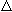H/ R whereH  is the heat of vaporization (the amount of heat necessary to convert 1.00 mole of a substance just below its boiling point to the gas phase) and R is the gas constant and for which, with the units we will be using, is equal to 8.31 x 10-3 kJ/mol K [recall that R = Nok (a mole's worth of k)• 4Consider the plenum type of vaporizer shown below in which a vaporizing chamber, which contains the volatile anesthetic liquid, is associated with a bypass system allowing a mixing of anesthetic vapor with anesthetic-free gas (diluent) which bypass is the chamber.

• This representation is quite simplistic relative to specific vaporizer designs; however it illustrates several principles that we have discussed so far.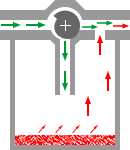• 4The saturated vapor pressure (SVP) describes the number of anesthetic molecules in the gas phase following equilibration between the carrier gas and liquid anesthetic.

• Those gases which are more highly volatile would be expected then to have a higher SVP.

• Therefore, anesthetics with a higher SVP would require a smaller ratio of the total gas flow into the vaporizer to pass through the actual vaporizing chamber in order to result in a given final anesthetic concentration delivered to the patient.

• 4The relationship between SVP and vaporizer flow relative to total flow is illustrated by comparing several volatile anesthetics with differing SVP values.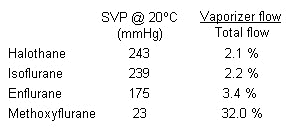• 4In comparing the extremes (halothane (Fluothane) with an SVP of 243 mm Hg with that of methoxyflurane within SVP of 23 mm Hg, the significant differences in the ratio vaporizer flow over total flow is apparent [2.1% versus 32%].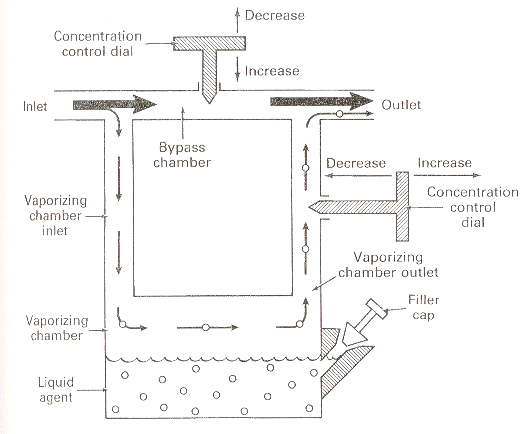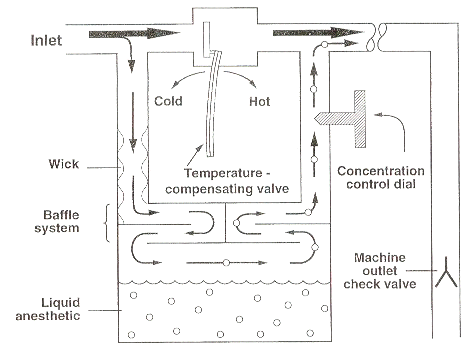4,,6SVP and Temperature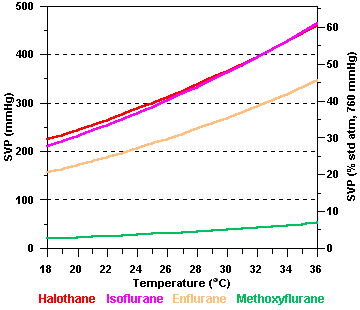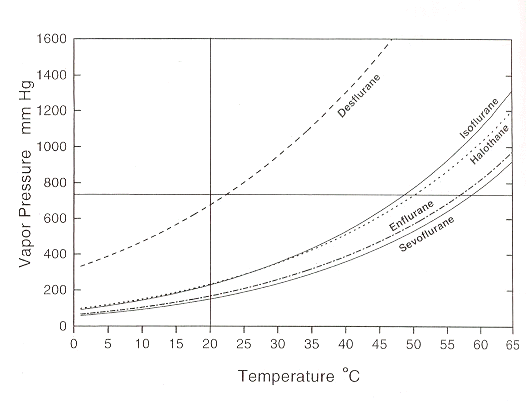• 4Note the variation in SVP as a function of temperature.  Accordingly, temperature compensation would be required in order to ensure that the vaporizer output would remain constant.  Such constancy requires that other variables are also held constant.  Some of these other variables include:

1. Changes in the composition of carrier gas which may alter total flow through the vaporizing chamber

2. Addition of nitrous oxide which may dissolve in the anesthetic volatile liquid component which may change the effective volume passing through the vaporizing chamber

3. Alteration in gas flow rate (if the flow rate of the gas through the vaporizing chamber is too high, effective equilibration may not be approached therefore the gas leaving the chamber will be relatively less saturated, resulting in a reduced vaporizer output)

4. Ambient pressure SVP is only temperature dependent; therefore if the ambient pressure were to be reduced, the ratio of the anesthetic vapor pressure to the total pressure would increase resulting in a higher output anesthetic concentration.

1. One example might be that if the halothane (Fluothane) vaporizer set (calibrated) for sea level (760 mm Hg) to deliver 2% anesthetic, the percent delivered in Denver (the mile high city) would be about 2.7 % halothane (Fluothane)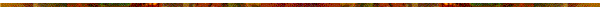• The equation that illustrates the relationship between vapor pressure and a given temperature and the heat of vaporization is the basis for  the Clausius-Clapeyron equation and has the general form ln(Pvap) = -Hvap/RT + b (compare this equation in terms of its form to the equation for a straight line, y = mx +b).

• If vapor  pressures were available in two different temperatures, into equations might be written:

1. ln(P2) =  -Hvap /RT2 + b and

2. ln(P1) =  -Hvap /RT1 + b

• Subtracting the first equation from the second equation and noting that the b's cancel, one obtains ln(P2) - ln(P1) = [-Hvap /R] *(1/T2-1/T1), which may be rewritten ln(P2/P1) =[ -Hvap /R]*(1/T2-1/T1) which is the Clausius-Clapeyron equation.• Example #1: The vapor pressure of water at 25 degrees C is about 24 mm Hg.  Furthermore the heat of vaporization is 40.7 kJ/mole.  What would be the vapor pressure of water at 97 degrees C.?

• To solve this problem, we can apply the Clausius-Clapeyron equation --[note that with the units chosen, R = 8.31 x 10-3 kJ/mole K.]

1.  ln(P2/P1) = -Hvap /R *(1/T2-1/T1)

2.  ln(P2/24mm Hg) = -40.7kJ/mole / 8.31 x 10-3 kJ/mole K *(1/370-1/298)

3. ln(P2/24mm Hg) = 3.198

4. P2/24mm Hg = 24.48

5. P2= 588 mmHg• Example #2: Another relationship which we will apply shortly to anesthetics would be the case in which we want to find out the heat of vaporization for a liquid, but we only know the vapor pressure at two different temperatures.  For this example the vapor pressure at 45 degrees C. is 100 mm Hg and the vapor pressure at 25 degrees C. is 46 mm Hg.  We would begin again with the Clausius-Clapeyron equation:

• Again as above, R = 8.31 x 10-3 kJ/mole K

1.  ln(P2/P1) = -Hvap /R *(1/T2-1/T1), rearranging

2. ln(100 mm Hg/46 mm Hg) = [-Hvap /8.31 x 10-3 kJ/mole K] *(1/318oK-1/298oK)

3. ln(100/46)*8.31 x 10-3 kJ/mole K /(1/318oK-1/298oK) = -Hvap OR

4. (0.776*0.00831 kJ/mole oK)/-2.11 x 10 -4oK = -Hvap

5. 30.56 kJ/mole =  -Hap

• 1Basic Physics and Measurement in Anaesthesia,   Davis, P.D., Parbrook, G.D. and Kenny G.N.C, 4th Edition, Butterworth Heinemann, pp 50-51, 1995.

• 2Basic Physics and Measurement in Anaesthesia,   Davis, P.D., Parbrook, G.D. and Kenny G.N.C, 4th Edition, Butterworth Heinemann, pp 54-55, 1995.

• 3Practical Anesthesia Physics: Part 1: Airway & Vaporizer, Rampil,I., 1995

• 4Anesthesia Equipment Resource (Anesthetic Vaporizers)

• 5Ebert, R. J. and Schmid III, P. G. "Inhalation Anesthesia" Ch. 15 in Clinical Anesthesia (Barash, P.G., Cullen, B. F., and Stoelting. R.K., eds) 4th Edition, Lippincott Williams& Wilkins, Philadelphia, pp. 377-387, 2001

• 5aPark, John L. "The Kinetic Molecular Theory of Ideal Gases.

• 6Andrews, J.J. and Brockwell, R. C "Delivery Systems for Inhaled Anesthetics" in Clinical Anesthesia (4/e) edited by Paul G. Barash, Bruce F. Cullen, and Robert K. Stoelting, Lippincott Williams& Wilkins, Philadelphia, pp. 574-575, 2001